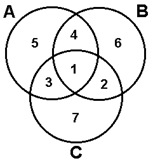CS420 Fall 2004 Homework, Week 5

In class we discussed hamming error correcting codes, in this homework we will explore an implementation of hamming (7,4) error correcting codes.

Review:

Hamming error correcting code of type (n, m) takes m bits of unencoded data and creates an n bit code. Hamming (7,4) code adds 3 error correction bits to 4 bits of data forming a 7 bit code such that any single bit error in the code may be corrected (Hamming (8,4) adds an overall parity bit that allows 2 bit errors to be detected and 1 bit errors corrected.

The algorithm may be described using the following Venn diagram:The first 4 bits of the hamming code (bits 1, 2, 3, 4 in the diagram) correspond to the 4 bits of data to encode. The next three bits (bits 5, 6, 7 in the diagram) are the error correcting bits and are computed so as to make the parity of the bits in each set (A, B, C) be even.

For this assignment assume little-endian bit and nybble (4 bit) ordering. This means that the least significant bit or nibble precedes higher order bits/nybbles.

EX: To compute the 1st ECC bit (bit 5 in the diagram) when encoding the data 1011 the data bits 1, 3, and 4 (1, 0, 1 respectively) would need to be set to 0 to maintain even parity since 1 ^ 0 ^ 1 = 0. Similarly, bit 6 would be computed by XORing data bits 1, 2, and 6 together and bit 7 is the even parity bit for set C.

To complete this assignment you will need to create five simple C programs: bitstream encoder, bitstream decoder, hamming encoder, hamming decoder, and a noise generator:

1.      A2B (ASCII to Bitstream Converter)

-          Inputs ASCII text on stdin

-          Outputs binary digits, ASCII 0’s and 1’s, to stdout

-          Input characters are processed one by one as they are received until EOF

-          Binary stream is little-endian (least significant bit first).

2.      Hamming (7,4) Encoder

-          Accepts an ASCII binary stream of 0’s and 1’s on stdin

-          Parses input stream into 4 bit data and outputs a stream of 7 bit hamming codes (using the algorithm described above) as ASCII 0’s and 1’s

-          Ends on EOF

-          Bit ordering should be maintained

3.      Hamming (7,4) Decoder

-          Inputs stream of ASCII 0’s and 1’s on stdin

-          Parses stream into 7 bit hamming codes and outputs decoded 4 bit data stream on stdout as ASCII 0’s and 1’s

-          Fix single bit errors by checking parity for each bit set, (A, B, C) in each hamming code by noting which set of set’s parity fails. Notice set intersection in the Venn diagram. EX: If only set C’s parity fails then bit 7 must be inverted. If parity fails for sets A and B (but not C) then bit 4 must be inverted. Or if parity fails for all sets then bit 1 is flipped.

4.      Noise generator

-          Accepts one floating point parameter between 0 and 1 indicating the probability of a given bit being corrupted (0.0 indicated a 0 probability of a bit flipping, 1 indicates that every bit will certainly be incorrect). Consider implementing this using a system random number generator creating random values between 0 and 1.0 then testing if the random value is less than the probability value.

-          Inputs a stream of ASCII 0 or 1 bits on stdin

-          Outputs stream of ASCII 0 or 1 bits on stout

-          Exits on EOF

5.      B2A (Bitstream to ASCII Converter)

-          Inputs ASCII 0’s and 1’s test on stdin

-          Parses input bit stream into 8 bit ASCII characters (assumes little endian bitstream) and outputs the resulting decoded characters on stdout

-          Stops on EOF

6.      Test

-          Test by piping the codecs together:

echo “One that makes you larger, and one that makes you small, and the ones that mother gives you don't do anything at all. Go ask Alice when she's ten feet tall.” | a2b | hamm | noise 0.0 | unhamm | b2a

-          Test at the following noise levels: 0, 0.01, 0.05, 0.1 and 0.5

Please submit the following for full credit:

-          Commented C code for each program

-          Each executable

-          Output from the 5 test runs (labeled to indicate which noise level)